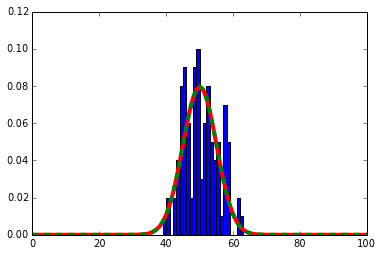# Binomial Distribution¶

## If I flip a coin 100 times, what is the probability of having exactly 42 heads?¶

In :
## simulate 100 trials of flipping a coin 100 times

num_trials = 100
num_flips = 100

target_number = 42
target_count = 0.

## for each trial
for t in xrange(num_trials):

## simulate a single trial of 100 flips
for f in xrange(num_flips):
r = random.randint(0,1)

## if the number of 0, record it as a head
if (r == 0):

target_count += 1

target_prob = target_count / num_trials
print "I saw %d %d of %d times for a probability of %0.5f" % (target_number, target_count, num_trials, target_prob)

I saw 42 2 of 100 times for a probability of 0.02000

In :
## print the number of times we saw heads in the first 50 trials

[62, 51, 51, 51, 42, 46, 45, 48, 46, 48, 46, 58, 44, 45, 49, 54, 61, 45, 49, 58, 46, 48, 57, 45, 57, 54, 52, 56, 52, 50, 43, 44, 61, 58, 44, 57, 49, 53, 45, 53, 55, 44, 50, 51, 55, 45, 45, 44, 51, 49]

In :
## Plot the histogram
#import matplotlib.pyplot as plt
plt.figure()
plt.show()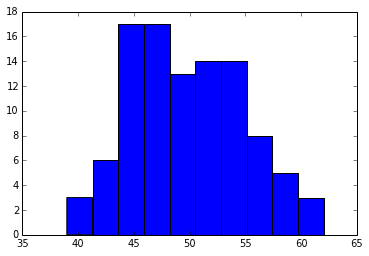In :
## Plot the histogram using integer values by creating more bins
plt.figure()
plt.title("Using integer values")
plt.show()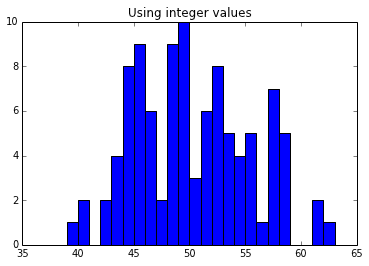In :
## Plot the density function, notice bars sum to exactly 1
plt.figure()
plt.show()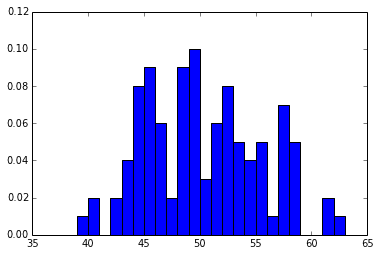In :
## Compute mean & stdev of distribution
mean = (sum(head_counts) + 0.) / num_trials
sumdiff = 0.0
diff = (count - mean) ** 2
sumdiff += diff

sumdiff /= num_trials
stdev = math.sqrt(sumdiff)

print "The average value was %0.2f +/- %0.2f" % (mean, stdev)

The average value was 49.81 +/- 5.17


## Can we figure out what the distribution should look like analytically?¶

Ah, the good old binomial distribution!

$$p(\text{k heads in n flips}) = {n \choose k} p^{k} (1-p)^{(n-k)}$$$$p \leftarrow \text { probability of heads in a single flip }$$$$p^k \leftarrow \text{ total probability of k heads}$$$$1-p \leftarrow \text{ probabilty of one tails}$$$$n-k \leftarrow \text{ number of tails}$$$$(1-p)^{(n-k)} \leftarrow \text{ probability of all the tails}$$$${n \choose k} \leftarrow \text{ all the different orderings of k heads in n flips}$$

Reminder:

$${n \choose k} = \frac{n!}{k!(n-k)!}$$
In :
## Compute the probability of seeing 42 heads
print "The probability of seeing 42 heads in %d trials is %.05f" % (num_trials, prob_42)

The probability of seeing 42 heads in 100 trials is 0.02229

In :
## evaluate the probability density function for the range [0, num_flips]
prob_dist = []
for k in xrange(num_flips+1):
prob_dist.append(prob_k)

plt.figure()
plt.plot(prob_dist, color='red', linewidth=4)
plt.show()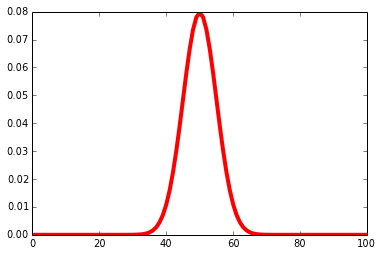In :
## overlay the analytic distribution over the data
plt.figure()
plt.plot(prob_dist, color='red', linewidth=4)
plt.show()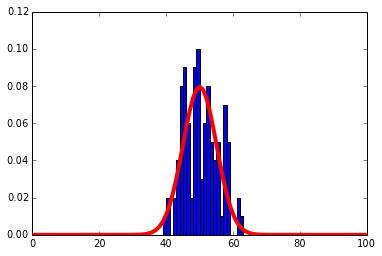mean = n * p

## FACT: The standard deviation of the binomial distribution is¶

$$stdev = \sqrt(np(1 − p))$$

(For a fixed probability, grows with the sqrt of the number of trials)

In :
expected_mean  = num_flips * prob_heads

print "The expected frequency is %.02f +/- %.02f" % (expected_mean, expected_stdev)
print "The observed frequency was %0.2f +/- %0.2f" % (mean, stdev)

The expected frequency is 50.00 +/- 5.00
The observed frequency was 49.81 +/- 5.17


## It is imprecise to compute the factorial of very large numbers, use the gaussian (normal) approximation instead¶

$$p(x)=\frac{1}{\sqrt{2 \pi \sigma^2}} \exp \left( -\frac{(x-\mu)^2}{2 \sigma^2} \right)$$

where $\sigma$ is the standard deviation and $\mu$ is the mean

This is nice because you can estimate the distribution in your head if you know the mean and standard deviationIn :
gaus_prob_dist = []
pi = 3.1415926

for k in xrange(num_flips+1):
var = expected_stdev**2
num = math.exp(-(float(k)-expected_mean)**2/(2*var))
denom = math.sqrt(2*pi*var)
gaus_prob = num/denom
gaus_prob_dist.append(gaus_prob)

plt.figure()
plt.plot(gaus_prob_dist, color='green', linewidth=4, linestyle='dashed')
plt.show()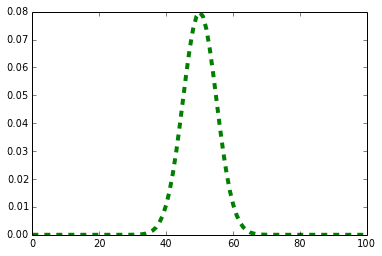In :
## overlay the analytic distributions over the data
plt.figure()